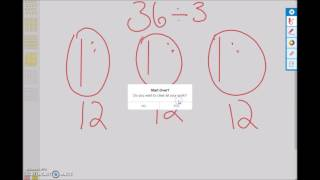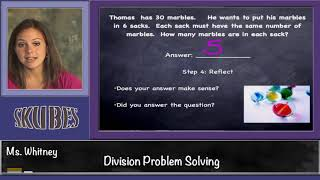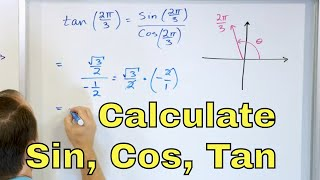# Division - Sharing Equally: Grade 3

##By university of Houston mathematics education

Use multiplication and division within 100 to solve word problems in situations involving equal groups, arrays, and measurement quantities, e.g., by using drawings and equations with a symbol for the unknown number to represent the problem.# Division - Sharing Equally: Grade 3

##By university of Houston mathematics education

Use multiplication and division within 100 to solve word problems in situations involving equal groups, arrays, and measurement quantities, e.g., by using drawings and equations with a symbol for the unknown number to represent the problem.# Division Problem Solving

##By Skubes ed

Use multiplication and division within 100 to solve word problems in situations involving equal groups, arrays, and measurement quantities, e.g., by using drawings and equations with a symbol for the unknown number to represent the problem.1# 08 - Calculate Sin, Cos & Tan w/ Unit Circle in Radians - Part 1

##By Math and Science

Quality Math And Science Videos that feature step-by-step example problems!